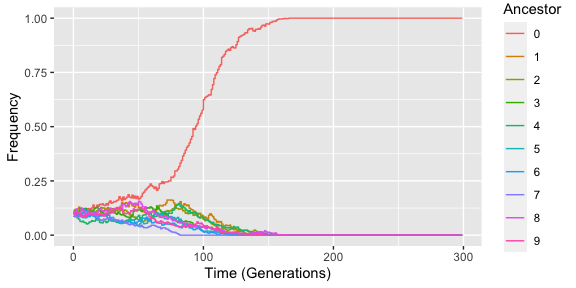# GenomeAdmixR

The package GenomeAdmixR was designed to simulate the generation of iso-female lines, and simulate genomic changes during, and after, formation of iso-female lines. Furthermore, it allows for simulation of effects after crossing individuals from iso-female lines.

library(GenomeAdmixR)
library(ggplot2)
packageVersion("GenomeAdmixR")
##  '2.1.1'

## Simulating an iso-female line

In order to create an iso-female line, we first have to create a ‘wild’ population, from which we will draw individuals. To create such a population, we make use of the function ‘simulate_admixture’, using the ancestry module:

wildpop <-  simulate_admixture(
module = ancestry_module(number_of_founders = 4,
morgan = 1),
pop_size = 100,
total_runtime = 1000)

This creates a population of 100 diploid individuals (e.g. 2N = 200), based upon 10 founders (e.g. 10 individuals that can be genetically distinguished from each other). Then, for 1000 generations, this population is inbred and genomes of the 20 founders are allowed to mix. All individuals have 2 chromosomes (for simplicity and computational speed, we only model one chromosome pair), which are 1 Morgan long. The result can be written to file (optional), which can be usefull when generating for instance an extremely large wild population, which can be used later (e.g. read from file).

The result of the function is a structure containing 1000 individuals:

wildpop
## $population ##  "Population with 100 individuals" ## ## attr(,"class") ##  "genomadmixr_simulation" Each individual has two chromosomes, with a given number of junctions (e.g. delineations between two contiguous genomic stretches from different ancestors): wildpop[] ##  "Population with 100 individuals" In order to create an iso-female line, two random individuals are drawn from the wild population, and selected for inbreeding. We can simulate this process with: isofemale <- create_iso_female( module = ancestry_module(input_population = wildpop, morgan = 1), n = 1, inbreeding_pop_size = 1000, run_time = 200) Here, n indicates the number of isofemales to be created from the same source population, the inbreeding population size is set to be small, in order to speed up computation. Maximum run time is best set hight, to assure that all individuals become genetically identical. # Visualizing individuals Now, we can plot the isofemales, this plots the two chromosomes next to each other, where colors indicate different ancestors: plot(isofemale[])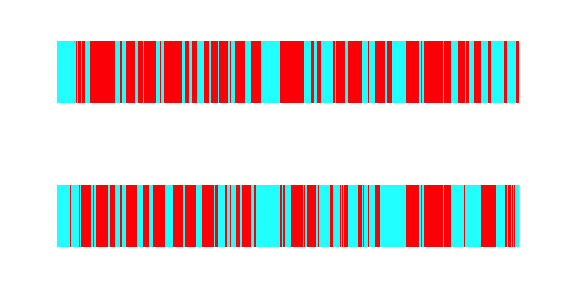Because we have chosen 4 ancestors, these plots are not terribly informative, let’s try a toy example: wildpop <- simulate_admixture( module = ancestry_module(number_of_founders = 4, morgan = 1), pop_size = 100, total_runtime = 100) isofemale <- create_iso_female( module = ancestry_module(input_population = wildpop, morgan = 1), n = 1, inbreeding_pop_size = 100, run_time = 10000) plot(wildpop$population[])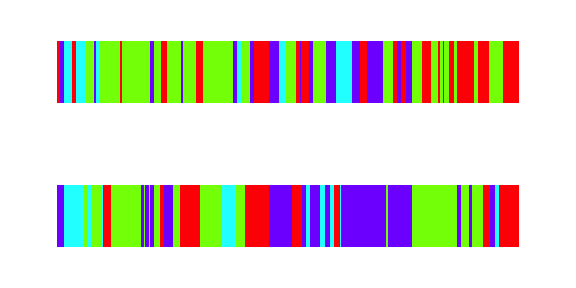plot(isofemale[])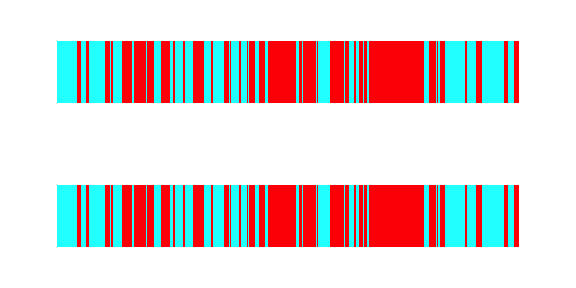We can also plot a (section of) a single chromosome:

plot_chromosome(isofemale[]$chromosome1, xmin = 0.0, xmax = 0.5)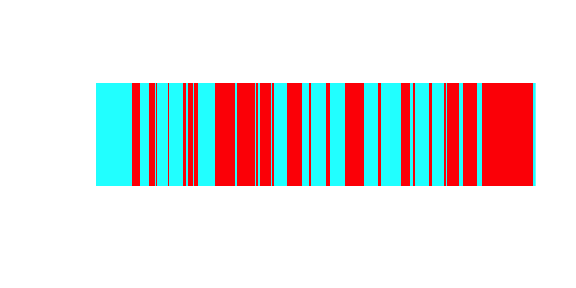# Multiple source populations It gets more interesting if we create two populations, and draw iso-females from them, and cross these. First, we have to create two populations. both_populations <- simulate_admixture( module = ancestry_module(morgan = 1), migration = migration_settings(population_size = c(100, 100), migration_rate = 0.0, initial_frequencies = list(c(rep(1, 20), rep(0, 20)), c(rep(0, 20), rep(1, 20))) ), total_runtime = 1000) ## Warning in check_initial_frequencies(initial_frequencies): starting frequencies were normalized to 1 ## Warning in check_initial_frequencies(initial_frequencies): starting frequencies were normalized to 1 population_1 <- both_populations$population_1

population_2 <- both_populations$population_2 To make sure that the two populations dont share ancestor indices, we use the function ‘increase_ancestor’. Now that we have two populations, we can generate two different iso-females from them: isofemales <- create_iso_female( module = ancestry_module(input_population = population_1, morgan = 1), n = 2, inbreeding_pop_size = 100, run_time = 10000) plot_chromosome(isofemales[]$chromosome1, 0, 1)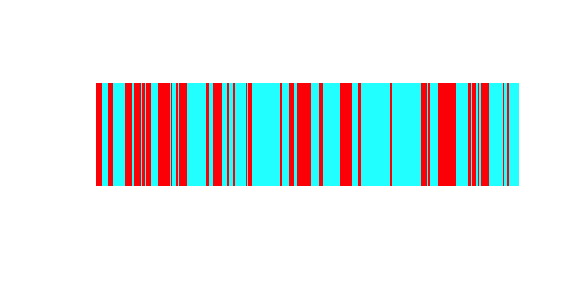plot_chromosome(isofemales[]$chromosome1, 0, 1)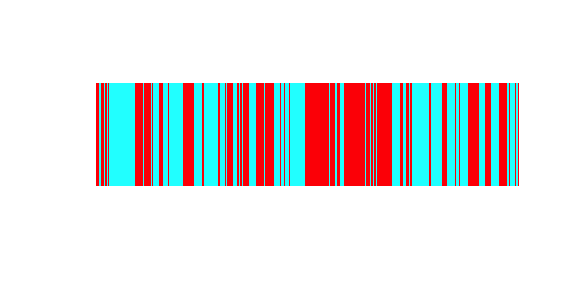We can now use these two isofemales to seed a new inbreeding population: mixed_population <- simulate_admixture( module = ancestry_module(input_population = list(isofemales[], isofemales[]), morgan = 1), pop_size = 100, total_runtime = 100) And plot some individuals from our new population: plot(mixed_population$population[])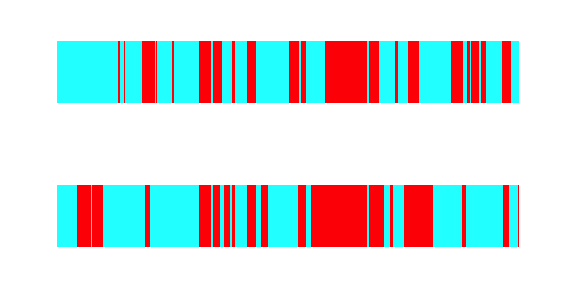# Statistics

## FST

We can show the effect of overlap by calculating the Fst value:

fst <- calculate_fst(population_1,
population_2,
sampled_individuals = 10,
number_of_markers = 100,
random_markers = TRUE)
fst
##  0.9936111

The FST calculation function uses the library hierfstat to calculate the FST. To do so, it samples 10 random individuals (the parameter sampled individuals) from the population, and then assesses the genetic content at 100 randomly placed markers (number of markers, and random_markers = TRUE). To increase power, a higher number of individuals can be sampled, although this does increase computational load.

## LD

We can also calculate LD statistics. LD is only defined for markers, so again we have to simulate artificial markers along the chromosome.

  ld_results <- calculate_ld(wildpop,
markers = 10)

plot(ld_results$ld_matrix~ld_results$dist_matrix,
xlab = "Genetic Distance (Morgan)",
ylab = "LD",
pch = 16)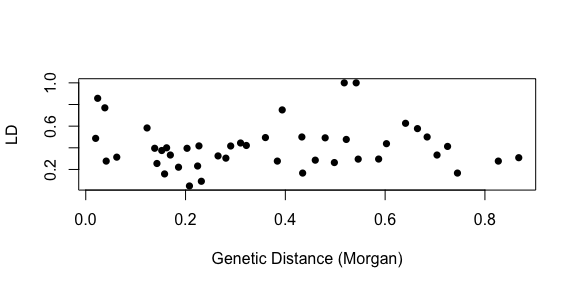plot(ld_results$rsq_matrix~ld_results$dist_matrix,
xlab = "Genetic Distance (Morgan)",
ylab = "r_sq",
pch = 16)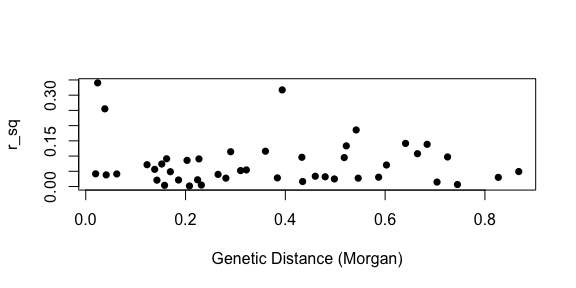plot(ld_results$ld_matrix~ld_results$rsq_matrix,
xlab = "r_sq",
ylab = "LD",
pch = 16)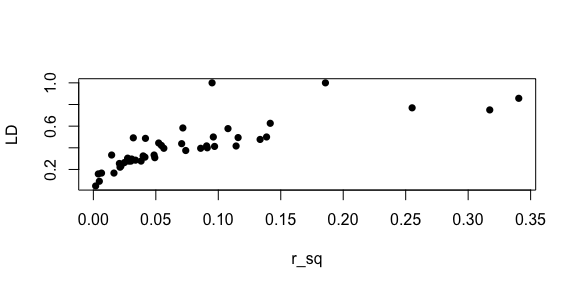To analyze LD patterns better, it makes more sense to create a population from scratch. We expect for a strongly inbred population, that there is no LD:

no_ld_pop <- simulate_admixture(
module = ancestry_module(number_of_founders = 4,
morgan = 1),
pop_size = 100,
total_runtime = 1000)

ld_results <- calculate_ld(no_ld_pop,
sampled_individuals = 10,
markers = 10)

plot(ld_results$ld_matrix~ld_results$dist_matrix,
pch = 16,
xlab = "Distance",
ylab = "LD",
xlim = c(0, 1),
ylim = c(0, 1))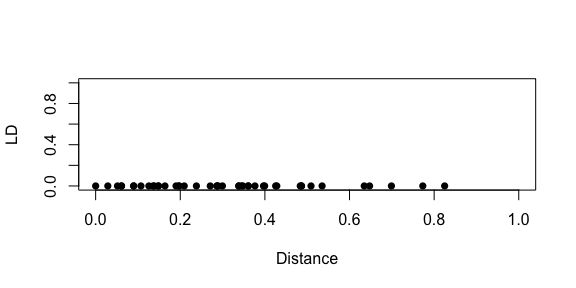Alternatively, for a barely inbred population, we expect a negative relationship between LD and distance:

strong_ld_pop <- simulate_admixture(
module = ancestry_module(number_of_founders = 4,
morgan = 1),
pop_size = 100,
total_runtime = 10)

ld_results <- calculate_ld(strong_ld_pop,
sampled_individuals = 10,
markers = 10)

plot(ld_results$ld_matrix~ld_results$dist_matrix,
pch = 16,
xlab = "Distance",
ylab = "LD",
xlim = c(0, 1),
ylim = c(0, 1))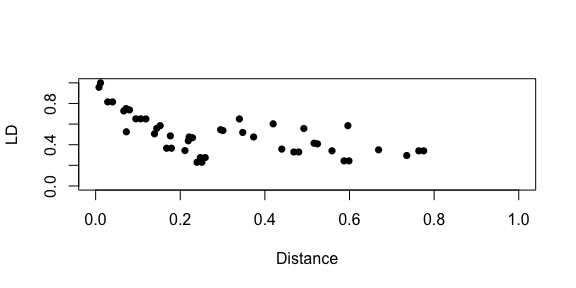# Selection

Selection for specific markers (e.g. SNPs) can potentially cause local allelel frequency biases, and influence genetic patterns within the population as a whole.

The user can provide a selection ‘matrix’ that specifies how selection acts upon different genotypes. As genotypes we indicate here ‘aa’ (wildtype), ‘aA’ (heterozygote) and ‘AA’ (homozygote with allele under selection), where wildtype is any allele not under selection. As alleles under selection we take the general genomic content of ancestors, and hence alleles refer to anestors.

A perhaps interesting simulation would be one where the heterozygote has an advantage over the other genotypes

s <- 0.1
selection_matrix <- matrix(nrow = 1, ncol = 5)
selection_matrix[1, ] <- c(0.5,
1.0, 1.0 + s, 1.0,
0)

markers <- 0.5

selected_pop <- simulate_admixture(
module = ancestry_module(number_of_founders = 2,
morgan = 1,
markers = markers),
pop_size = 1000,
total_runtime = 100,
select_matrix = selection_matrix)
## Found a selection matrix, performing simulation including selection
plot_over_time(selected_pop$frequencies, markers)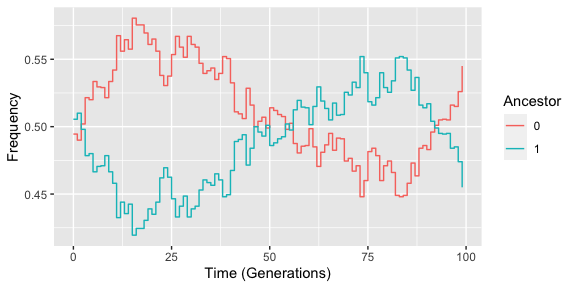Indeed we observe that the frequencies of both ancestors are in equilibrium around 0.5, as expected. Another interesting simulation would be where we give only the homozygous mutant a selective benefit. Here we expect that it takes some time before the homozygote takes over. s <- 0.1 selection_matrix[1, ] <- c(0.5, 1.0, 1.0, 1.0 + s, 0) selected_pop <- simulate_admixture( module = ancestry_module(number_of_founders = 10, morgan = 1, markers = markers), pop_size = 1000, total_runtime = 300, select_matrix = selection_matrix) ## Found a selection matrix, performing simulation including selection plot_over_time(selected_pop$frequencies, markers)